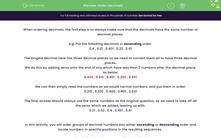# Order Decimals

In this worksheet, students will practice ordering decimals into ascending or descending sequences using place value.Key stage:  KS 4

Year:  GCSE

GCSE Subjects:   Maths

GCSE Boards:   Pearson Edexcel, OCR, Eduqas, AQA,

Curriculum topic:   Number, Fractions, Decimals and Percentages

Curriculum subtopic:   Structure and Calculation Ordering Fractions, Decimals and Percentages

Popular topics:   Decimals worksheets

Difficulty level:#### Worksheet Overview

When ordering decimals, the first step is to always make sure that the decimals have the same number of decimal places.

e.g. Put the following decimals in ascending order:

0.4 , 0.21 , 0.401 , 0.32 , 0.61

The longest decimal here has three decimal places so we need to convert them all to have three decimal places.

We do this by adding zeros onto the end of any which have less than 3 numbers after the decimal place, as below:

0.400 , 0.210 , 0.401 , 0.320 , 0.610

We can then simply read the numbers as we would normal numbers, and put them in order:

0.210 , 0.320 , 0.400 , 0.401 , 0.610

The final answer should always use the same numbers as the original question, so we need to take off all the zeros which we added, leaving us with:

0.21 , 0.32 , 0.4 , 0.401 , 0.61

In this activity, you will order groups of decimal numbers into either ascending or descending order and locate numbers in specific positions in the resulting sequences.

Now let's attempt some questions together!

### What is EdPlace?

We're your National Curriculum aligned online education content provider helping each child succeed in English, maths and science from year 1 to GCSE. With an EdPlace account you’ll be able to track and measure progress, helping each child achieve their best. We build confidence and attainment by personalising each child’s learning at a level that suits them.

Get started